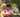### CSS transform property

December 26, 2019

CSS transform property

The transform property applies a 2D or 3D transformation to an element. This property allows you to rotate, scale, move, skew, etc., elements.

### Syntax

``transform: none|transform-functions|initial|inherit;``
ValueDescription
noneDefines that there should be no transformation
matrix(n,n,n,n,n,n)Defines a 2D transformation, using a matrix of six values
matrix3d (n,n,n,n,n,n,n,n,n,n,n,n,n,n,n,n)Defines a 3D transformation, using a 4x4 matrix of 16 values
translate(x,y)Defines a 2D translation
translate3d(x,y,z)Defines a 3D translation
translateX(x)Defines a translation, using only the value for the X-axis
translateY(y)Defines a translation, using only the value for the Y-axis
translateZ(z)Defines a 3D translation, using only the value for the Z-axis
scale(x,y)Defines a 2D scale transformation
scale3d(x,y,z)Defines a 3D scale transformation
scaleX(x)Defines a scale transformation by giving a value for the X-axis
scaleY(y)Defines a scale transformation by giving a value for the Y-axis
scaleZ(z)Defines a 3D scale transformation by giving a value for the Z-axis
rotate(angle)Defines a 2D rotation, the angle is specified in the parameter
rotate3d(x,y,z,angle)Defines a 3D rotation
rotateX(angle)Defines a 3D rotation along the X-axis
rotateY(angle)Defines a 3D rotation along the Y-axis
rotateZ(angle)Defines a 3D rotation along the Z-axis
skew(x-angle,y-angle)Defines a 2D skew transformation along the X- and the Y-axis
skewX(angle)Defines a 2D skew transformation along the X-axis
skewY(angle)Defines a 2D skew transformation along the Y-axis
perspective(n)Defines a perspective view for a 3D transformed element

The translate() method moves an element from its current position (according to the parameters given for the X-axis and the Y-axis).

The rotate() method rotates an element clockwise or counter-clockwise according to a given degree. Using negative values will rotate the element counter-clockwise.

The scale() method increases or decreases the size of an element (according to the parameters given for the width and height).

The skew() method skews an element along the X and Y-axis by the given angles.

The matrix() method combines all the 2D transform methods into one. The matrix() method take six parameters, containing mathematic functions, which allows you to rotate, scale, move (translate), and skew elements.Posted by Tai Lu ( 呂台生 ) who's building useful things.You should follow him on Twitter